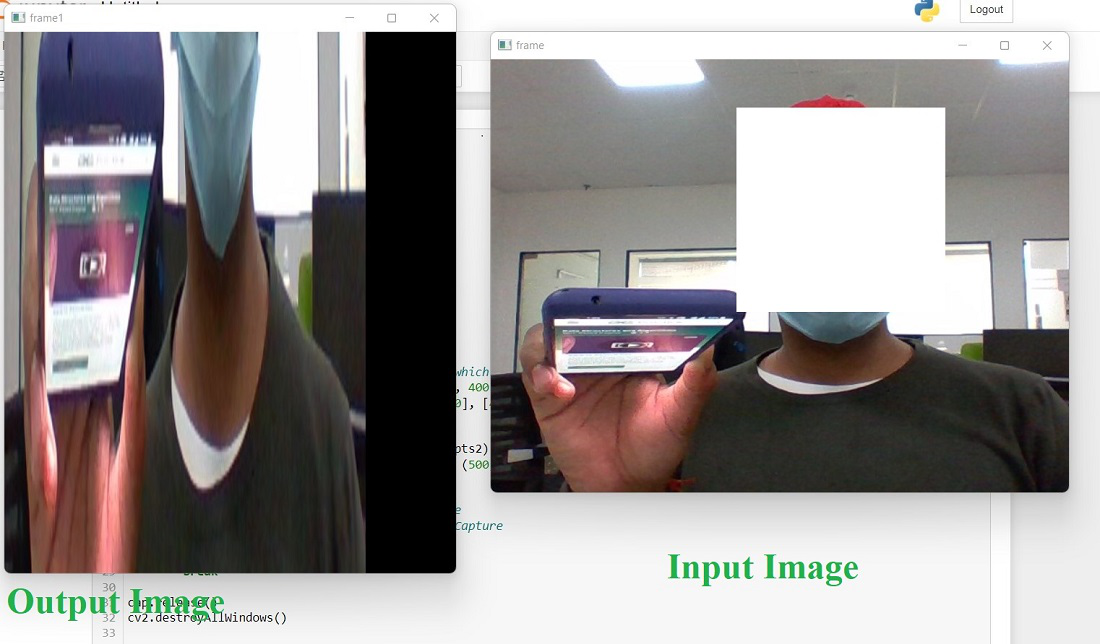Open in App
Not now

# Perspective Transformation – Python OpenCV

• Difficulty Level : Easy
• Last Updated : 03 Jan, 2022

In Perspective Transformation, we can change the perspective of a given image or video for getting better insights into the required information. In Perspective Transformation, we need to provide the points on the image from which want to gather information by changing the perspective. We also need to provide the points inside which we want to display our image. Then, we get the perspective transform from the two given sets of points and wrap it with the original image.

We use cv2.getPerspectiveTransform and then cv2.warpPerspective .

cv2.getPerspectiveTransform method

Syntax: cv2.getPerspectiveTransform(src, dst)

Parameters

• src: Coordinates of quadrangle vertices in the source image.
• dst: Coordinates of the corresponding quadrangle vertices in the destination image.

cv2.wrapPerspective method

Syntax: cv2.warpPerspective(src, dst, dsize)

Parameters

• src: Source Image
• dst: output image that has the size dsize and the same type as src.
• dsize: size of output image

Below is the Python code explaining Perspective Transformation:

## Python3

 `# import necessary libraries `   `import` `cv2 ` `import` `numpy as np `   `# Turn on Laptop's webcam` `cap ``=` `cv2.VideoCapture(``0``)`   `while` `True``:` `    `  `    ``ret, frame ``=` `cap.read()`   `    ``# Locate points of the documents` `    ``# or object which you want to transform` `    ``pts1 ``=` `np.float32([[``0``, ``260``], [``640``, ``260``],` `                       ``[``0``, ``400``], [``640``, ``400``]])` `    ``pts2 ``=` `np.float32([[``0``, ``0``], [``400``, ``0``],` `                       ``[``0``, ``640``], [``400``, ``640``]])` `    `  `    ``# Apply Perspective Transform Algorithm` `    ``matrix ``=` `cv2.getPerspectiveTransform(pts1, pts2)` `    ``result ``=` `cv2.warpPerspective(frame, matrix, (``500``, ``600``))` `    `  `    ``# Wrap the transformed image` `    ``cv2.imshow(``'frame'``, frame) ``# Initial Capture` `    ``cv2.imshow(``'frame1'``, result) ``# Transformed Capture`   `    ``if` `cv2.waitKey(``24``) ``=``=` `27``:` `        ``break`   `cap.release()` `cv2.destroyAllWindows()`

Output:My Personal Notes arrow_drop_up
Related Articles